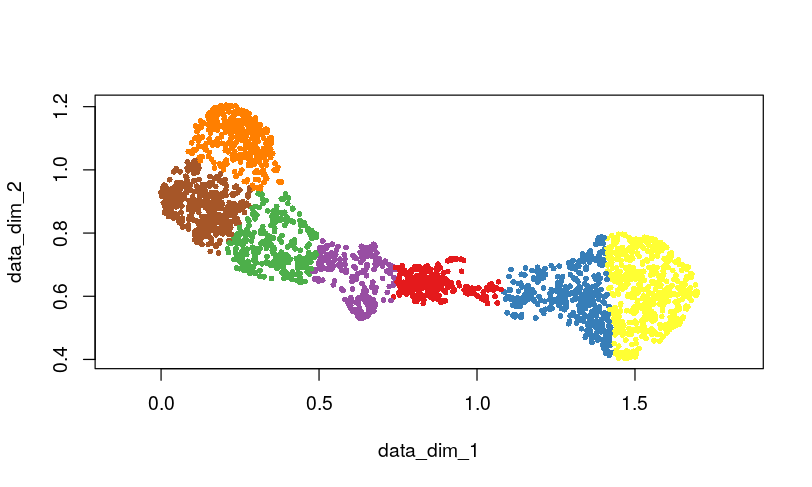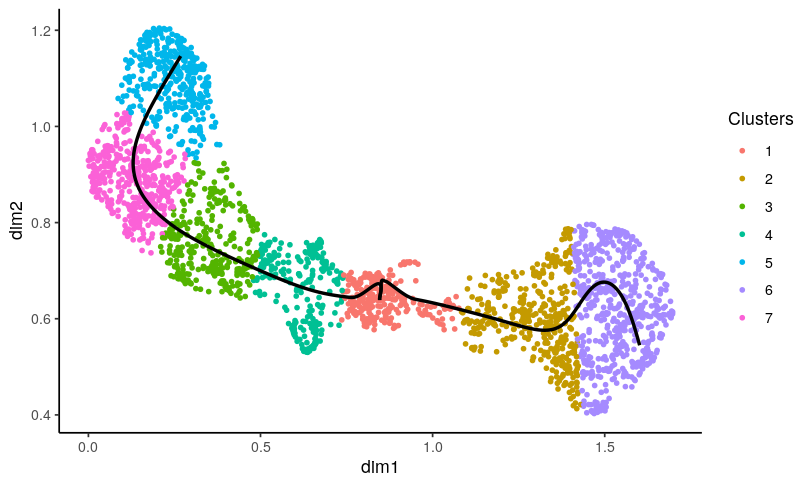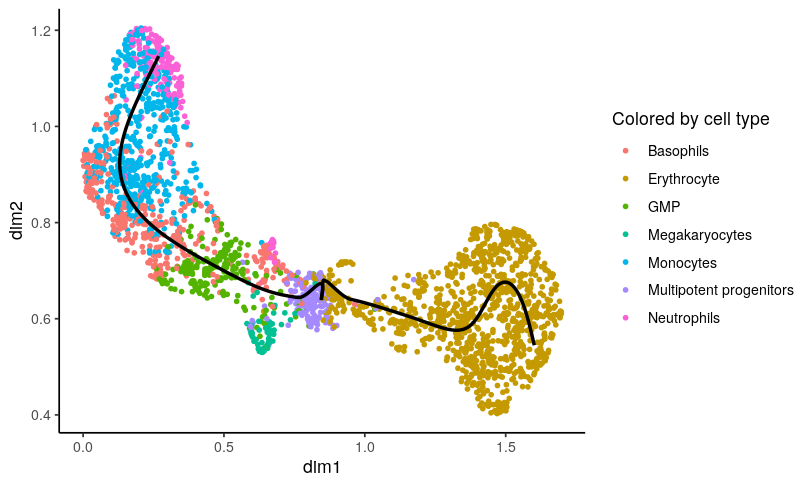# Introduction

`tradeSeq` is an `R` package that allows analysis of gene expression along trajectories. While it has been developed and applied to single-cell RNA-sequencing (scRNA-seq) data, its applicability extends beyond that, and also allows the analysis of, e.g., single-cell ATAC-seq data along trajectories or bulk RNA-seq time series datasets. For every gene in the dataset, `tradeSeq` fits a generalized additive model (GAM) by building on the `mgcv` R package. It then allows statistical inference on the GAM by assessing contrasts of the parameters of the fitted GAM model, ading in interpreting complex datasets. All details about the `tradeSeq` model and statistical tests are described in our preprint (Van den Berge et al. 2019).

In this vignette, we analyse a subset of the data from (Paul et al. 2015). A `SingleCellExperiment` object of the data has been provided with the `tradeSeq` package and can be retrieved as shown below. The data and UMAP reduced dimensions were derived from following the Monocle 3 vignette.

# Installation

To install the package, simply run

``````if(!requireNamespace("BiocManager", quietly = TRUE)) {
install.packages("BiocManager")
}

``````library(tradeSeq)
library(RColorBrewer)
library(SingleCellExperiment)
library(slingshot)

# For reproducibility
RNGversion("3.5.0")
palette(brewer.pal(8, "Dark2"))
counts <- countMatrix
rm(countMatrix)

# Fit trajectories using slingshot

We will fit developmental trajectories using the `slingshot` package (Street et al. 2018). `slingshot` requires cluster labels as input, and fits trajectories in reduced dimension. We will use the reduced space calculated with the UMAP method, which is pre-calculated in the `se` object. We cluster the data using k-means with \(7\) clusters. Since we know which cells are the progenitor cell type, we define the starting point for the trajectories as input for `slingshot`. Note that this argument is optional, and not required to run `slingshot`.

``````set.seed(200)
rd <- reducedDims(crv)
cl <- kmeans(rd, centers = 7)\$cluster
plot(rd, col = brewer.pal(9, "Set1")[cl], pch = 16, asp = 1,
cex = 2/3)````````lin <- getLineages(rd, clusterLabels = cl, start.clus = 1)``
``## Using full covariance matrix``
``crv <- getCurves(lin)``

We find two lineages for this dataset. The trajectory can be visualized using the `plotGeneCount` function, using either the cluster labels or cell type to color the cells.

``plotGeneCount(curve = crv, clusters = cl)````````plotGeneCount(curve = crv, clusters = celltype,
title = "Colored by cell type")``````# Determining the number of knots

After estimating the trajectory, we can fit generalized additive models (GAMs) with the `tradeSeq` package. Internally, this package builds on the `mgcv` package by fitting additive models using the `gam` function. The core function from `tradeSeq`, `fitGAM`, will use cubic splines as basis functions, and it tries to ensure that every lineage will end at a knot point of a smoother. By default, we allow for \(6\) knots for every lineage, but this can be changed with the `nknots` argument. More knots will allow more flexibility, but also increase the risk of overfitting.

Ideally, the number of knots should be selected to reach an optimal bias-variance trade-off for the smoother, where one explains as much variability in the expression data as possible with only a few regression coefficients. In order to guide that choice, we developed diagnostic plots using the Akaike Informaction Criterion (AIC). This is implemented in the `evaluateK` function in `tradeSeq`. The function takes as input the expression count matrix, a `SlingshotDataSet` (or alternatively, a matrix of pseudotimes and cell-level weights). The range of knots to evaluate is provided with the `knots` argument. The minimum allowed number of knots is \(3\). While there is no boundary on the maximum number of knots, typically the interesting range is around \(3\) to \(10\) knots. The function will fit NB-GAM models for some number of genes, provided by the `nGenes` argument, over the range of knots that are provided, and return the AIC for each gene fitted with each number of knots. It is generally a good idea to evaluate this multiple times using different seeds (using the `set.seed` function), to check whether the results are reproducible across different gene subsets.

This task can be computationally demanding, since the models must be fit multiple times for each gene. We therefore skip this in the vignette, but show the output one can expect instead.

``````icMat <- evaluateK(counts = counts, sds = crv, k=3:20, nGenes = 200,
verbose=FALSE)
# alternatively:
icMat <- evaluateK(counts = counts, k=3:20, nGenes = 200,
pseudotime = slingPseudotime(crv, na = FALSE),
cellWeights = slingCurveWeights(crv))``````